# Core Java. Lecture #6

## Before generics

``````Manager ceo = ...;
Manager cto = ...;
Employee cleaner = ...;
List managers = new ArrayList();

//bug!!

//typecast with runtime exception -- too late!
Manager m = (Manager) managers.get(2);``````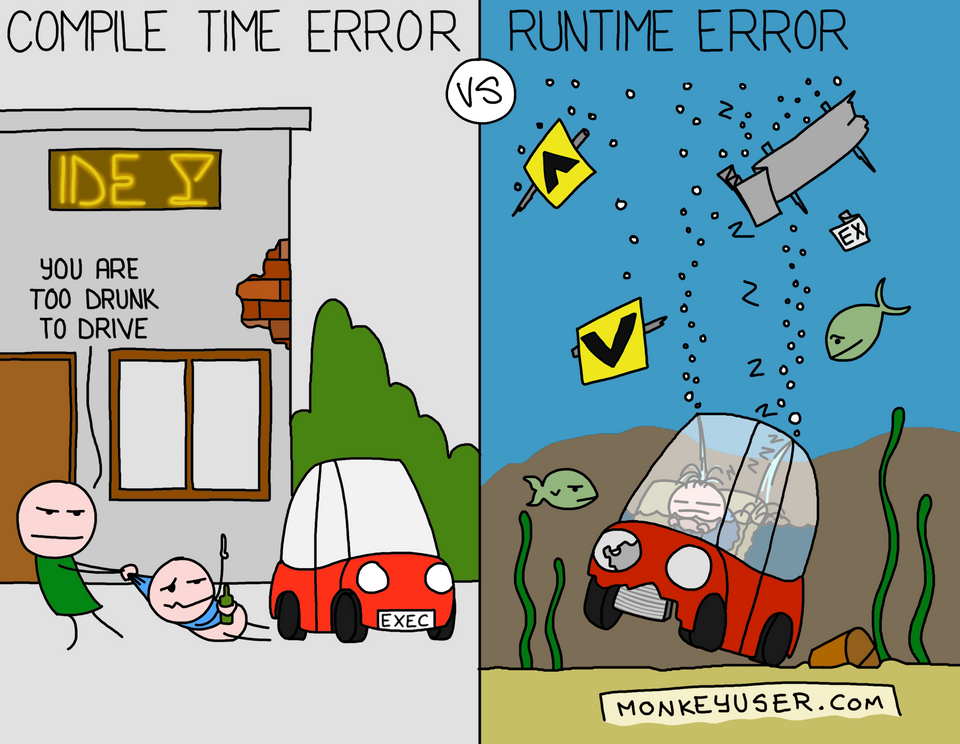## After generics

``````Manager ceo = ...;
Manager cto = ...;
Employee cleaner = ...;
List<Manager> managers = new ArrayList<>();

// won't compile!

// no type casting is needed!
Manager m = managers.get(1);``````

## Defining our own parameterized class

``````public class Pair<T> {
private T first;
private T second;
public Pair() { first = null; second = null; }
public Pair(T first, T second) {
this.first = first;
this.second = second;
}
public T getFirst() { return first; }
public T getSecond() { return second; }
public void setFirst(T newValue) { first = newValue; }
public void setSecond(T newValue) { second = newValue; }
}``````

## Definition and use

``````Pair<String> pair = new Pair<>("John", "Mary");

//Which is equivalent to replacing T with String
Pair(String, String)
String getFirst()
String getSecond()
void setFirst(String)
void setSecond(String)``````

## Generic methods

``````public <T> T getRandomItem(T... items) {
}

String s = getRandomItem("A", "B", "C");
Manager m = getRandomItem(ceo, cto, cfo);``````

## Another example

``````public <T> T getRandomItem(List<T> items) {
T result =
items.get(
return result;
}``````

## Intermediate conclusions

• Using parameterized classes is simple (just specify parameters, `List<Manager>`)

• Using parameterized methods is even simpler: type inference: `Manager m = getRandomItem(…​);`

• Writing your own parameterized classes and methods is a more complex task.

## Bounded types

``````public <T extends Person> String getRandomPersonName(List<T> items) {
Person result = //you can write T result = … as well

return result.getName();
}``````

## Intersection types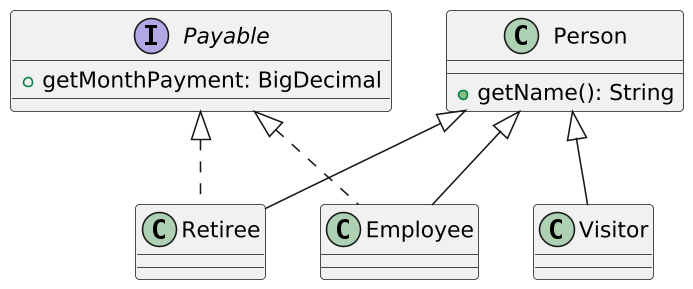``````//you can add with ampersand as many interfaces as you like,
//but not more than one class
public <T extends Person & Payable>
String getRandomNameAndPayment(List<T> items) {
T result =
items.get(
return result.getName() //from Person!
+ result.getPayment(); //from Payable!
}``````

## Implementation of generics

• Appeared in Java 5

• There was a problem of backward compatibility

• Generic classes is a language capability, not a platform capability

• Type Erasure, damn it!

## Raw types

 Generic Type (source) Raw Type (compiled) ``````class Pair { private T first; private T second; Pair(T first, T second) {this.first = first; this.second = second;} T getFirst() {return first; } T getSecond() {return second; } void setFirst(T newValue) {first = newValue;} void setSecond(T newValue) {second = newValue;} }`````` ``````class Pair { private Object first; private Object second; Pair(Object first, Object second) {this.first = first; this.second = second;} Object getFirst() {return first; } Object getSecond() {return second; } void setFirst(Object newValue) {first = newValue;} void setSecond(Object newValue) {second = newValue;} }``````

## Bounded types instead of Object

 Generic Type (source) Raw Type (compiled) ``````class Pair{ private T first; private T second; Pair(T first, T second) {this.first = first; this.second = second;} T getFirst() {return first; } T getSecond() {return second; } void setFirst(T newValue) {first = newValue;} void setSecond(T newValue) {second = newValue;} }`````` ``````class Pair { private Employee first; private Employee second; Pair(Employee first, Employee second) {this.first = first; this.second = second;} Employee getFirst() {return first; } Employee getSecond() {return second; } void setFirst(Employee newValue) {first = newValue;} void setSecond(Employee newValue) {second = newValue;} }``````

## Method calls

 Source code Compiled ``````Pair buddies = new Pair<>(); /*type control in compile time*/ buddies.setFirst(cfo); buddies.setSecond(cto); /*type cast is not needed*/ Manager buddy = buddies.getFirst();`````` ``````Pair buddies = new Pair(); /*type control is not needed -- everything was checked at compile time!*/ buddies.setFirst(cfo); buddies.setSecond(cto); /*type cast inserted by compiler*/ Manager buddy = (Manager) buddies.getFirst();``````

## Bridge methods to preserve polymorphism

 Source code Compiled ``````class DateInterval extends Pair { @Override void setSecond( LocalDate second){ if (second .compareTo(getFirst())>=0){ super.setSecond(second); } } }`````` ``````class DateInterval extends Pair { void setSecond( LocalDate second){ if (second .compareTo(getFirst())>=0){ super.setSecond(second); } } //bridge method!! @Override void setSecond(Object second){ setSecond((LocalDate) second); } }``````

## Summary: how it works

• There are no parameterized classes in the JVM, only regular classes and methods.

• Type parameters are replaced with Object or with boundary type.

• Bridge methods are added to preserve polymorphism.

• Type cast is added as needed.

## Never use raw types

• The ability to use raw types as variable types is reserved for backward compatibility with code written before Java5.

• Java5 was released in 2004.

``````List<Manager> a = new ArrayList <> ();
//Raw type usage.
List b = a;
//Compiled and executed, the list is corrupted!
//Executed: list.get returned String as Object
System.out.println(b.get(0));
//ClassCastException on execution
Manager m = a.get(0);``````

Understanding generics in Java is not about what you can do with them, but about what you can’t do with them.

## Type Erase → unable to determine a type parameter in the runtime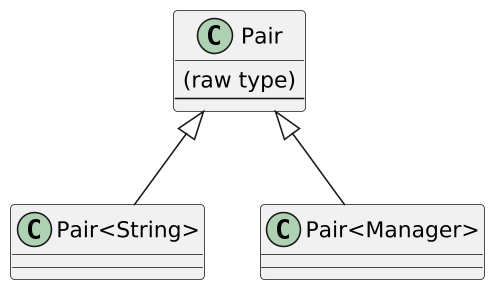``````//compilation error! we don't know the type parameter in the runtime!
if (a instanceof Pair<String>) ...

//that's how it goes...
if (a instanceof Pair<?>) ...``````

## Erasing types to Object → not being able to use primitive types as parameters

``````//alas, impossible!
List<int>  integers = ... //compilation error!

List<Integer> integers = ...
int v = integers.get(0); /*unboxing under the hood:
v = integers.get(0).intValue();*/``````

## Generics and primitives

• Today: Need performance? — we write special implementations.

• In the Standard Library: `Stream<Integer>``IntStream` `Stream<Double>``DoubleStream`.

• In specialized libraries like fastutil: `ArrayList<Integer>``IntArrayList`, `HashMap<Integer, V>``Int2ObjectMap<V>` (WARNING: the real need for such libraries is rare!!)

• Tomorrow: Project Valhalla, specialized generics. Will solve the problem once and for all.

## Parameter types cannot be instantiated

``````class Pair<T> {

T newValue {
return new T(); //alas, compilation error!
}
}``````

## Solved by metaclass and reflection (which we will talk about it later)

``````class Container<T> {
//  bounded wildcard type, to be explained later
Class <? extends T> clazz;

Container(Class<? extends T> clazz) {
this.clazz = clazz;
}

T newInstance() throws ReflectiveOperationException {
//if there is an accessible constructor without parameters!
return clazz.newInstance();
}
}

Container<Employee> container1 = new Container<>(Employee.class);``````

## Moreover, you cannot instantiate a type-parameter array

``````public T[] toArray(){
//Won't compile
return new T[size];
}``````

It is solved by passing a parameter, for example, to ArrayList:

``````/* If the array is of sufficient size - use it,
if it's too small - we construct a new one via reflection*/
public <T> T[] toArray(T[] a)``````

## Arrays and generics are enemies

``````//Won't compile: Generic Array Creation.
List<String>[] a = new ArrayList<String>;
//...because such an array will not have
//the full type information about its elements!``````

## Hammer the values into an array with a sledgehammer and make a heap pollution

``````List<String>[] a = (List<String>[])new List <?> ;
Object[] objArray = a;

objArray =  List.of("foo");

a = List.of(new Manager()); //won't compile!

objArray =  List.of(new Manager()); //will compile and run!

//Runtime error: Manager cannot be cast to String
String s = a.get(0);
//...and this is what is called heap pollution.``````

## Varargs — still an array…​

The same heap pollution as in the previous example:

``````static void dangerous(List<String>... stringLists){
List<Integer> intList = List.of(42);
Object[] objects = stringLists;
objects = intList;
ClassCastException
String s = stringLists.get(0);
}</Integer></String>

dangerous(new ArrayList<>());``````

## The compiler anticipates something…​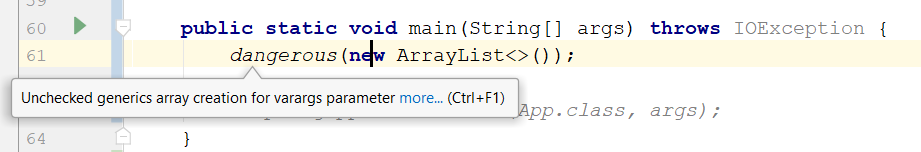To calm the compiler down, you need to put the annotation `@SafeVarargs`:

``````/*I solemnly promise I will not
do heap pollution!*/
@SafeVarargs
static void dangerous(List<String>...``````

…​and the compiler will calm down.

## Why?!

• That’s because having varargs with parameterized types is convenient!..

• `List.of(E…​ a)`

• `Collections.addAll(Collection<? super T> c, T…​ elements)`

• `EnumSet.of(E first, E…​ rest)`

• If you behave well, you can use `@SafeVarargs`, and everything will be fine:

• Do not write anything to the elements of the array,

• Do not pass a reference to the array of parameters to the outside methods.

## You cannot parameterize

• Exceptions

• catching exceptions is checking their types,

• raw types is everything we can check in the runtime.

• Anonymous classes

• Instantiated in place, there cannot be multiple classes parameterized differently.

• Enums.

## Type parameters cannot be used in a static context

``````public class Container<T> {
private static T value; //will not compile.
public static T getValue(); //will not compile
}

//Static context is one for all
Container<Foo>.getValue();
Container<Bar>.getValue();``````

## You cannot implement different parameterizations of the same interface in one class

 Source code Compiled ``````class Employee implements Comparable{ @Override int compareTo(Employee e){ ... } } class Manager extends Employee implements Comparable { @Override int compareTo(Manager m){ ... } }`````` ``````class Manager extends Employee implements Comparable{ //bridge method for Employee int compareTo(Object m) { return compareTo((Manager) m); } //bridge method for Manager int compareTo(Object e) { return compareTo((Employee) e); } //CLASH!!! }``````

## Array covariance vs generic invariance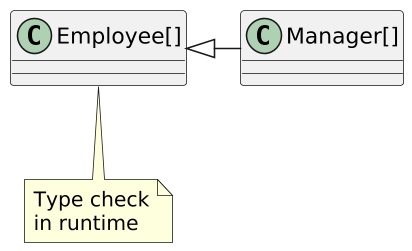``````Manager[] managers = ... Employee lowlyEmployee = ... Employee[] e = managers; /*ArrayStoreException in runtime*/ e = lowlyEmployee;``````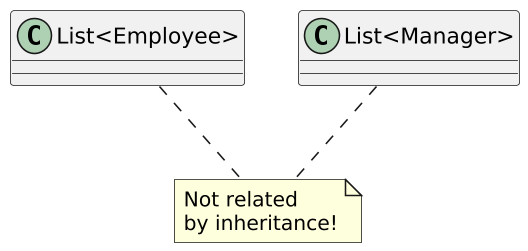``````List managers = ... Employee lowlyEmployee = ... /*Just won't compile*/ List e = managers;``````

## Real picture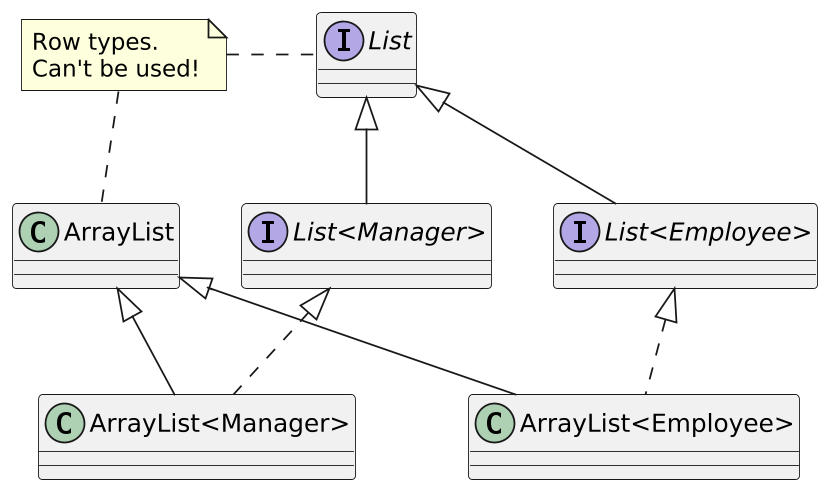## What if you want this?``````MyList<Manager> managers = ...
MyList<Employee> employees = ...

//Valid options, we want these to be compilable!

//Invalid options, we don't want these to be compilable!

## It won’t work this way…​

``````//only identically typed lists can be copied
class MyList<E> implements Iterable<E> {
void add(E item) { ... }
for (E item : list) this.add(item);
}
for (E item : this) list.add(item);
}
...}
MyList<Manager> managers = ...;  MyList<Employee> employees = ...;

## Java Covariant Wildcard Types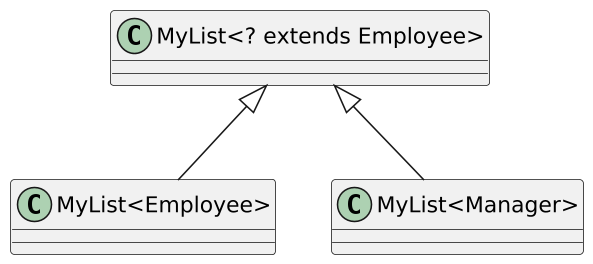``````class MyList<E> implements Iterable<E> {
//MyList<Manager> will do as a MyList<Employee>!!
void addAllFrom (MyList<? extends E> list){
}
}``````

## What can be done with an object typed `? extends`?

``````List<? extends E> list = ...

//it's understandable
E e1 = list.get(...);

E e2 = ...;
//won't compile! WHY??
//will compile. WHY??

In general, `addAllTo` will not work using `? extends`…​

## The opposite case (contravariant types)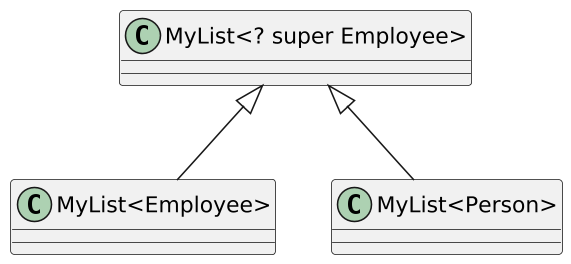``````class MyList<E> implements Iterable<E> {
//MyList<Person> will do as MyList<Employee>!!
void addAllTo (MyList<? super E> list) {
}
}``````

## What can be done with an object typed `? super`?

``````List<? super E> list = ...

E e1 = ...;
//will compile!

//Just Object. WHY??
Object e2 = list.get(...);``````

## Unbounded wildcard

• `List<?>` — is the same as `List<? extends Object>`. (Question: why not `<? super Object>`?)

• We can read elements, but only as `Object`.

• We can put only `null`s.

## Mnemonic rule

PECS

Producer Extends, Consumer Super

``````public static <T> T max (Collection<? extends T> coll,
Comparator<? super T> comp)

Collections.max(List<Integer>, Comparator<Number>)

Collections.max(List<String>, Comparator<Object>)``````

## Rules for using wildcard types

• Used in method arguments and local variables.

• Should not be returned. Their goal is to accept the arguments that need to be accepted and to reject the arguments that need to be rejected.

• Must be used in the API, otherwise the API will be too "oburate" and unusable.

## Wildcard Capture

``````public static void swap(Pair<?> p) {
Object f = p.getFirst();
Object s = p.getSecond();
//Oops!
// (we know that they have the correct type,
//  but there's nothing we can do)
p.setFirst(...);
p.setSecond(...);
}``````

## Method with type capture

``````public static void swap(Pair<?> p) {
swapHelper(p);
}

private static <T> void swapHelper(Pair<T> p) {
T f = p.getFirst();
p.setFirst(p.getSecond());
p.setSecond(f);
}``````

## Recursive Generics

``````class Holder<E, SELF extends Holder<E, SELF>>{
E value;
SELF setValue(E value){
this.value = value;
return (SELF) this;
}
}

class StringHolder extends Holder<String, StringHolder> {
void doSmth() {...};
}

new StringHolder().setValue("aaa").doSmth();``````

## Nada Amin & Rose Tate’s example

``````class Unsound {
static class Constrain<A, B extends A> {}
static class Bind<A> {
<B extends A>
A upcast(Constrain<A,B> constrain, B b) {
return b;
}
}
static <T,U> U coerce(T t) {
Constrain<U,? super T> constrain = null;
Bind<U> bind = new Bind<U>();
return bind.upcast(constrain, t);
}
public static void main(String[] args) {
String zero = Unsound.<Integer,String>coerce(0);
}
}``````

``````class Sample {